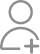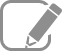Take Class 11 Tuition from the Best Tutors

•Affordable fees
•1-1 or Group class
•Flexible Timings
•Verified Tutors

Search in

# A three-wheeler starts from rest, accelerates uniformly with 1 m s–2 on a straight road for 10 s, and then moves with uniform velocity. Plot the distance covered by the vehicle during the nth second (n = 1,2,3….) versus n. What do you expect this plot to be during accelerated motion : a straight line or a parabola ?Follow 1AnswerStraight line Distance covered by a body in nth second is given by the relation Where, u = Initial velocity a = Acceleration n = Time = 1, 2, 3, ..... ,n In the given case, u = 0 and a = 1 m/s2 This relation shows that: Dn ∝ n … (iii) Now, substituting different values of n in equation...

Straight line

Distance covered by a body in nth second is given by the relationWhere,

u = Initial velocity

a = Acceleration

= Time = 1, 2, 3, ..... ,n

In the given case,

u = 0 and a = 1 m/s2This relation shows that:

Dn ∝ … (iii)

Now, substituting different values of n in equation (iii), we get the following table:

 n 1 2 3 4 5 6 7 8 9 10 Dn 0.5 1.5 2.5 3.5 4.5 5.5 6.5 7.5 8.5 9.5

The plot between and Dn will be a straight line as shown:Since the given three-wheeler acquires uniform velocity after 10 s, the line will be parallel to the time-axis after n = 10 s.

Now ask question in any of the 1000+ Categories, and get Answers from Tutors and Trainers on UrbanPro.com

Find Class 11 Tuition near you

Looking for Class 11 Tuition ?

Learn from the Best Tutors on UrbanPro

Are you a Tutor or Training Institute?

Join UrbanPro Today to find students near you
X

### Looking for Class 11 Tuition Classes?

The best tutors for Class 11 Tuition Classes are on UrbanPro

• Select the best Tutor
• Book & Attend a Free Demo
• Pay and start Learning### Take Class 11 Tuition with the Best Tutors

The best Tutors for Class 11 Tuition Classes are on UrbanPro